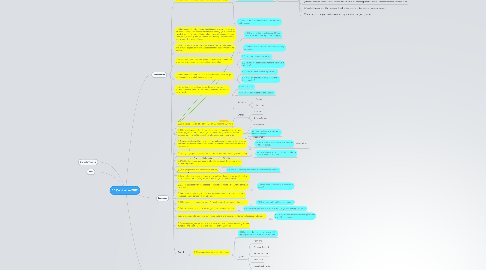# 1:1 Curriculum 2012

JW
John Wu
Get Started. It's Free1:1 Curriculum 2012 by## 1. Mathematics

### 1.1. (U) Mathematics is the science of patterns and relationships

1.1.1. (Q) Why is recognising patterns useful?

1.1.2. (Q) How can we describe relationships?

1.1.2.1. Relationships

1.1.2.1.1. (1) directly proportional (one quantity always keeps the same proportion to another)

1.1.2.1.2. (2) inversely proportional (as one quantity increases, the other decreases proportionally)

1.1.2.1.3. (3) accelerated (as one quantity increases uniformly, the other increases faster and faster)

1.1.2.1.4. (4) converging (as one quantity increases without limit, the other approaches closer and closer to some limiting value)

1.1.2.1.5. (5) cyclical (as one quantity increases, the other increases and decreases in repeating cycles)

1.1.2.1.6. (6) stepped (as one quantity changes smoothly, the other changes in jumps)

1.1.3. (Q) What is the difference between patterns and relationships?

### 1.2. (U) Mathematical Inquiry is to express ideas or to solve problems by: (1) representing some aspects of things abstractly, (2) manipulating the abstractions by rules of logic to find new relationships between them, and (3) seeing whether the new relationships say something useful about the original things

1.2.1. (Q) How is mathematical inquiry different from other inquiries? eg. scientific inquiry

### 1.3. (U) The process of abstraction is to observe a similarity between two or more objects or events and representing the common using symbols

1.3.1. (Q) What is the purpose of abstracting through symbols?

### 1.4. (U) After abstraction, symbols can be combined and recombined in various ways according to precisely defined rules

1.4.1. (Q) What are mathematical rules?

1.4.2. (Q) How do we determine whether a rule is valid or logical?

### 1.5. (U) Mathematical Inquiry can lead to a model from which insights can be gained or predictions can be made

1.5.1. (Q) How are these models represented?

1.5.2. (Q) What are the real world applications of these models?

### 1.6. (U) All mathematics is related as set theory in that every mathematical statement or proof could be cast into formulas within a set

1.6.1. (Q) What is a set?

1.6.2. (Q) How are sets related to one another?

### 1.7. Structure

1.7.1. Pure Mathematics

1.7.1.1. Study of:

1.7.1.1.1. Structure

1.7.1.1.2. Change

1.7.1.1.3. Space

1.7.1.1.4. Quantity

1.7.2. Practical Mathematics

1.7.2.1. Probability

1.7.2.2. Statistics

1.7.2.3. Sampling

## 3. Language

### 3.2. (U) Human language is highly complex in that it is based on a set of rules relating symbols to their meanings, thereby forming an infinite number of possible innovative utterances from a finite number of elements

3.2.1. (Q) How can language be used in different contexts?

### 3.3. (U) Spoken and signed languages contain a phonological system that governs how sounds or visual symbols are used to form sequences known as words or morphemes

3.3.1. (Q) What are the phenomes that make up a certain language?

### 3.4. (U) Many languages use stress, pitch, duration and tone to distinguish meaning

3.4.1. (Q) How does stress, pitch, duration and tone express different meaning?

### 3.6. (U) Languages evolve and diversify over time,

3.6.1. (Q) How has the language evolve in the Singapore context?

### 3.8. (U) All languages rely on the process of semiosis to relate a sign with a particular meaning.

3.8.1. (Q) How does semiotics give meaning to words?

### 3.10. (U) Humans use language as a way of signalling identity with one cultural group

3.10.1. (Q) How does culture affect its language?

### 3.11. (U) words are built of several meaningful units called morphemes

3.11.1. (Q) How does prefixes, infixes, suffixes change the meaning of a word?

### 3.12. (U) a syntactic system governs how words and morphemes are used to form phrases and utterances

3.12.1. (Q) What are the syntax/rules that govern the use of the language?

### 3.14. English

3.14.1. (U) Language is determined by its genre

3.14.1.1. (Q) How does the purpose of the use of the language determine its structure and tone?

3.14.1.2. genre

3.14.1.2.1. Narrative

3.14.1.2.2. Personal Recount

3.14.1.2.3. Factual Recount

3.14.1.2.4. Persuasive

3.14.1.2.5. Evaluation & Review

3.14.1.2.6. Procedure